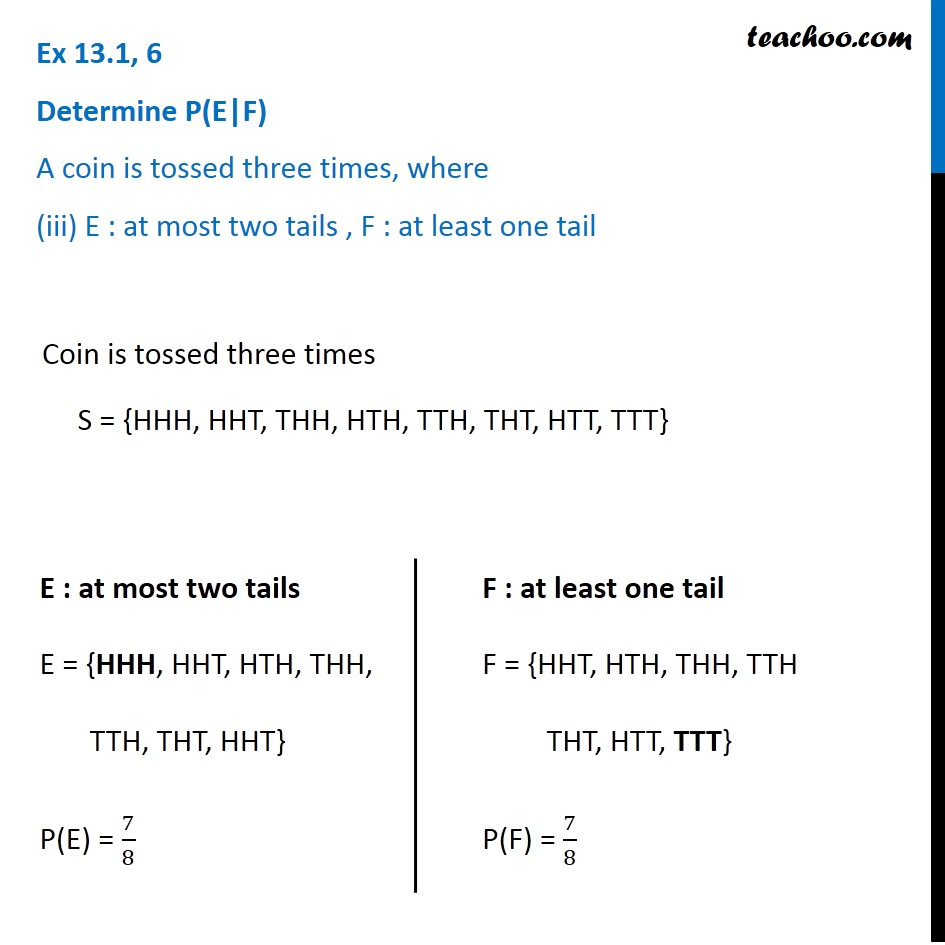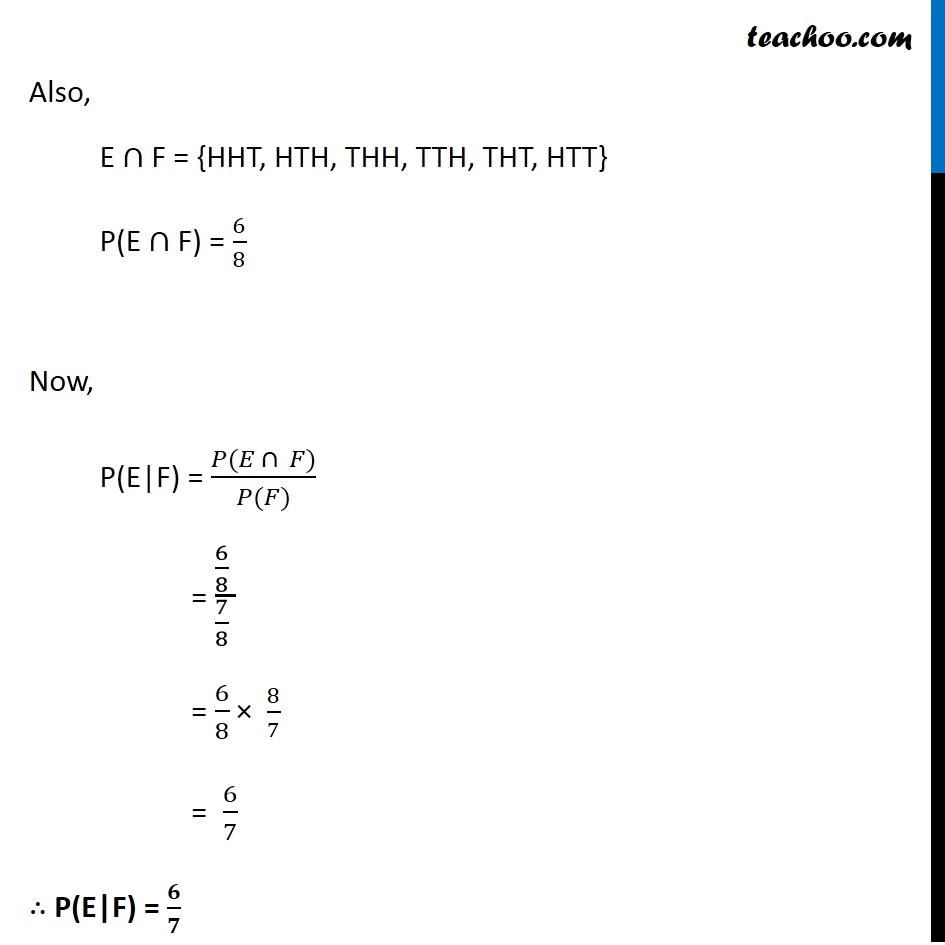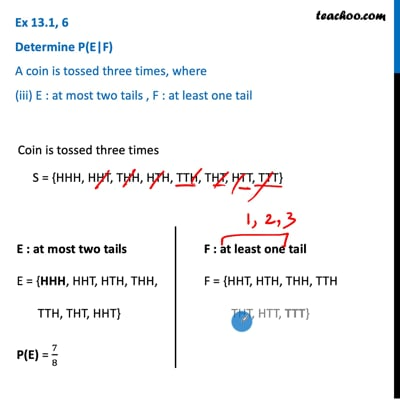Ex 13.1

Chapter 13 Class 12 Probability
Serial order wiseThis video is only available for Teachoo black users

Maths Crash Course - Live lectures + all videos + Real time Doubt solving!

### Transcript

Ex 13.1, 6 ﻿Determine P(E|F) A coin is tossed three times, where (iii) E : at most two tails , F : at least one tail Coin is tossed three times S = {HHH, HHT, THH, HTH, TTH, THT, HTT, TTT} E : at most two tails E = {HHH, HHT, HTH, THH, TTH, THT, HHT} P(E) = 7/8 F : at least one tail F = {HHT, HTH, THH, TTH THT, HTT, TTT} P(F) = 7/8 Also, E ∩ F = {HHT, HTH, THH, TTH, THT, HTT} P(E ∩ F) = 6/8 Now, P(E|F) = (𝑃(𝐸 ∩ 𝐹))/(𝑃(𝐹)) = (6/8 )/(7/8 ) = 6/8 × 8/7 = 6/7 ∴ P(E|F) = 𝟔/𝟕